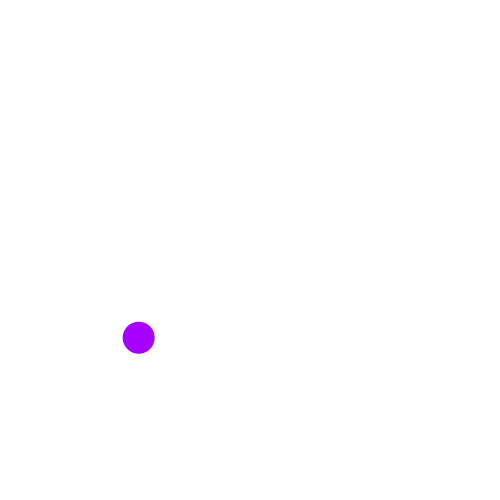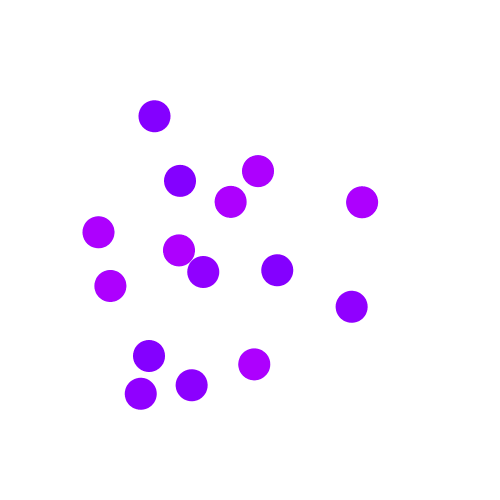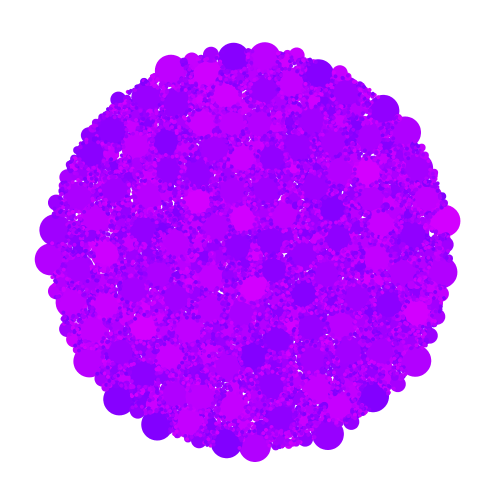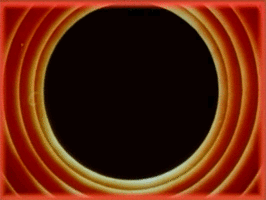# Circle Packing Using Stochastic Search

All Processing code for this article, along with images and animated GIFs, can be found on Github

In this post I’m going to explain the idea behind stochastic search and a few examples of how it can be applied to circle packing.

“Stochastic search” is just a fancy way of saying that we’re going to perform a random search until some condition is met. This is particularly useful for problems where a systematic approach is far too computationally expensive.

Now we’re interested in stochastic search within the context of generative art where the biggest application is in packing shapes. The basic algorithm for stochastic search looks like this:

Start with an initial state
while (some exit condition is not met) {
Generate a random candidate solution
if (It is a valid solution in the current state) {
Great! We now have a new state for the next iteration.
}
}


This might seem vague at this point, so let’s actually implement some examples in Processing so we can play around with it.

## Circle Packing

We’re going to apply stochastic search to the problem of packing circles of different sizes into a specific area.

Let’s start with a simple Circle class. Each circle has an (x, y) coordinate that specifies its center, and a radius r.

class Circle {
float x;
float y;
float r;

Circle(float x, float y, float r) {
this.x = x;
this.y = y;
this.r = r;
}

void draw() {
noStroke();
colorMode(HSB, 360, 100, 100);
fill(280 + random(-10, 10), 100, 100);
ellipse(x, y, 2*r, 2*r);
}
};


In the above code, we define two methods for our Circle class: a constructor to initialize a new object and a draw() method that draws it onto the screen using a random color.

We then define an ArrayList of Circle objects that we’re going to add to every time we find a “valid” circle to place on the screen.

ArrayList<Circle> circles;

void setup() {
size(500, 500);
circles = new ArrayList<Circle>();
background(255);
}


Now we get to the draw() function which serves as one iteration of our stochastic search loop. Recall that what we want to do is (i) generate a random candidate solution, (ii) check if it’s valid, and (iii) trigger some kind of exit condition so the sketch stops at some point.

Here is a simple version below:

int failed_tries = 0;

void draw() {
Circle nc = new Circle(random(width), random(height), 16);
if (isValidCircle(nc)) {
nc.draw();
} else {
failed_tries++;
if (failed_tries > 16 * 1024) {
println("Done!");
noLoop();
}
}
}


In the above code, we first create a Circle object at a random location on screen, with some fixed radius (16 pixels). We then check if this circle satisfies certain conditions that we want using a yet-undefined isValidCircle() function. If it turns out to satisfy all our conditions, and isValidCircle() returns true, we draw this circle to the screen and add it to the list of all valid circles (you’ll see in a bit why we need this array in the first place).

If, on the other hand, some condition wasn’t met and isValidCircle() returned false, we increment a counter (failed_tries, initially set to 0) specifying how many unsuccessful tries we’ve had. If the number of failed tries exceeds some threshold (in the above code, I arbitrarily picked 16 * 1024 tries), then we call the noLoop() function which ends the sketch.

Now, we of course still need to define our isValidCircle() method! What should go in this? Well, it depends on what you want! Let’s me give you some examples to show you what I mean.

### Tightly Packing Circles

Suppose we want to tightly pack circles, and when I say tightly packed, I just mean that we allow circles to be touching, placed right next to each other.

So what should our isValidCircle() function look like for this? Here it is:

class Circle {
...
boolean collides(Circle c) {
if (dist(x, y, c.x, c.y) < (r + c.r))
return true;
return false;
}
...
};

boolean isValidCircle(Circle new_circle) {
for (Circle c : circles)
if (new_circle.collides(c))
return false;

return true;
}


Let’s first look at the isValidCircle() function itself. Recall that the parameter new_circle that is provied as a parameter is the randomly-create circle in our draw() function. We iterate over every currently-valid circle (see why we need the circles list now?) and if any of them collides with our circle, we return false. Otherwise, if the loop completes without any problems, which means no current circle collides with the newly-created one, we return true.

Now let’s look at the collides() function we add to the Circle class. It takes as a parameter a circle, and checks if this circle collides with it. How do we check if two circles collide? It’s easy: if the distance between their centers is less than the sum of their radii, then they collide! We use Processing’s dist() function here.

And that’s it! Here’s how it looks, although it takes much longer to actually generate because of the failed tries. On my machine it took around 15 minutes! In general, the higher you set the threshold for failed_tries, the better a packing you get, but the longer it takes.### Packing Circles with Margins

Suppose we want to add some margins to separate circles by a small distance.

We can simply modify our collides() function to account for this! All we do is account for it in our distance calculation. We only consider a circle to collide with another if the distance between their centers is greater than the sum of their radii and a user-specified margin (in the MARGIN variable below).

int MARGIN = 10;

class Circle {
...
boolean collides(Circle c) {
if (dist(x, y, c.x, c.y) < (r + c.r + MARGIN))
return true;
return false;
}
...
};


And here is the result. Note how the circles are no longer touching and are separated by a margin of at least 10 pixels!### Packing Circles of Different Size

We can also modify our loop to pack circles of different sizes!

We do this by reducing the radius of the randomly-created circle when the number of failed tries at a given radius exceeds some threshold. Here is an example loop I wrote for doing this.

float RADIUS_MAX = 16;
int failed_tries = 0;

void setup() {
...
...
}

void draw() {
Circle nc = new Circle(random(width), random(height), current_radius);
if (isValidCircle(nc)) {
...
} else {
failed_tries++;

if (failed_tries > 32 * 1024 / current_radius) {
failed_tries = 0;
println("Done!");
noLoop();
}
}
}
}


In the above loop, the first change we make is that we create circles based on a radius stored in a current_radius variable (which is initialized to RADIUS_MAX in setup()).

Second we modify the exit condition of our loop. Recall that earlier we called noLoop() when the number of failed tried exceeded a threshold. Instead, now when we exceed a threshold, we reduce current_radius by half and reset failed_tries to zero.

Next, we change our threshold for failed_tries to be dependent on the current radius. Why? The reason is that we want more tries when the radius is smaller since the screen will already be packed with bigger circles, making it harder to find a valid circle.

Finally, we still need to finish the sketch at some point. In this case, we end the sketch if we hit our threshold of failed tries and the new, halved radius is less than a specified minimum radius (RADIUS_MIN).

And that’s it! We now have a circle packing algorithm that looks like this:### Packing Inside a Shape

The approach is also very flexible in that isValidCircle() can be modified to account for other conditions, such as whether it is inside a custom shape.

Here’s a simple version of isValidCircle() that additionally checks if it inside a radius of 200 pixels from the center of the screen (see the first two lines).

boolean isValidCircle(Circle nc) {
if (dist(nc.x, nc.y, width/2, height/2) > 200)
return false;

for (Circle c : circles)
if (nc.collides(c))
return false;

return true;
}


Of course, in order to improve this, we can also be a little smarter about how we generate our random circles, but I leave that to you as an exercise (read: I’m lazy!).

And here is the result:Really, this can be extended to account for any condition you want. For example, you can check if the circle is inside a polygon (see here for Tyler Hobbs’ polygonContainsPoint() function)

### Allowing Overlapping Circles

Here is another example showing the flexibility of this simple algorithm. We set the margin between circles to a negative number, therefore allowing the overlapping of circles.

int MARGIN = -5;


And here is the result!I also did one with a much longer run to get the following image.You can even try to modify the exit condition so that it stops the sketch when no more white pixels remain on the screen, although it would take significantly longer.

## Next Steps

You can try implementing similar stuff for rectangles or arbitrary polygons. In a future post, we’ll also look at how we can speed this up significantly using spacial search with a QuadTree. Stay tuned!

In the meantime, you can subscribe to my list to stay informed on new posts! No spam, guaranteed.